# Replace missing value with a subgroup-calculated value

Member Posts: 5Newbie
Suppose we have rows of data with attributes Temp & City & a Datetime (amongst others).  The temp data has some missing values.

How can I fill in the missing temp data with the average temp of the given city on an hourly basis?

Calculate average temp by City by datetime to the hour
Use that average temp to fill in any missing temp values for the corresponding City and datetime within the same hour.

Thoughts?
Tagged:

• Member Posts: 5Newbie
edited September 24
An acceptable alternative would be to a solution to fill in the missing temp by finding a non-missing temp value for the same City and Hour as the current row.
• Member Posts: 59Guru
Hi @TimT, given you have datetime data, you could perhaps try windowing your dataset by each hour using Process Windows, and using the 'Replace Missing Values (Series)' operator with the replace type numerical parameter set to average. Also have the 'ensure finite values' tickbox on here. I've built a small example on the Lake Heuron dataset which substitutes the ten year average into missing values. Hopefully this should help.

<?xml version="1.0" encoding="UTF-8"?><process version="10.2.000">
<context>
<input/>
<output/>
<macros/>
</context>
<operator activated="true" class="process" compatibility="10.2.000" expanded="true" name="Process" origin="GENERATED_TUTORIAL">
<parameter key="logverbosity" value="init"/>
<parameter key="random_seed" value="2001"/>
<parameter key="send_mail" value="never"/>
<parameter key="process_duration_for_mail" value="30"/>
<parameter key="encoding" value="SYSTEM"/>
<process expanded="true">
<operator activated="true" class="retrieve" compatibility="10.2.000" expanded="true" height="68" name="Retrieve Lake Huron" origin="GENERATED_TUTORIAL" width="90" x="112" y="34">
<parameter key="repository_entry" value="//Samples/Time Series/data sets/Lake Huron"/>
</operator>
<operator activated="true" class="blending:generate_columns" compatibility="10.2.000" expanded="true" height="82" name="Generate Attributes" origin="GENERATED_TUTORIAL" width="90" x="246" y="34">
<list key="function_descriptions">
<parameter key="data with missing values" value="if(rand()&lt;0.1,MISSING_NUMERIC,[Lake surface level / feet])"/>
</list>
<parameter key="keep_all_columns" value="true"/>
</operator>
<operator activated="true" class="parse_numbers" compatibility="10.2.000" expanded="true" height="82" name="Parse Numbers" width="90" x="380" y="34">
<parameter key="attribute_filter_type" value="all"/>
<parameter key="attribute" value=""/>
<parameter key="attributes" value=""/>
<parameter key="use_except_expression" value="false"/>
<parameter key="value_type" value="nominal"/>
<parameter key="use_value_type_exception" value="false"/>
<parameter key="except_value_type" value="file_path"/>
<parameter key="block_type" value="single_value"/>
<parameter key="use_block_type_exception" value="false"/>
<parameter key="except_block_type" value="single_value"/>
<parameter key="invert_selection" value="false"/>
<parameter key="include_special_attributes" value="false"/>
<parameter key="decimal_character" value="."/>
<parameter key="grouped_digits" value="false"/>
<parameter key="grouping_character" value=","/>
<parameter key="infinity_representation" value=""/>
<parameter key="unparsable_value_handling" value="fail"/>
</operator>
<operator activated="false" class="time_series:windowing" compatibility="10.1.000" expanded="true" height="103" name="Windowing" width="90" x="179" y="340">
<parameter key="attribute_filter_type" value="all"/>
<parameter key="attribute" value="Date"/>
<parameter key="attributes" value=""/>
<parameter key="use_except_expression" value="false"/>
<parameter key="value_type" value="attribute_value"/>
<parameter key="use_value_type_exception" value="false"/>
<parameter key="except_value_type" value="time"/>
<parameter key="block_type" value="attribute_block"/>
<parameter key="use_block_type_exception" value="false"/>
<parameter key="except_block_type" value="value_matrix_row_start"/>
<parameter key="invert_selection" value="false"/>
<parameter key="include_special_attributes" value="true"/>
<parameter key="has_indices" value="false"/>
<parameter key="indices_attribute" value="Date"/>
<parameter key="sort_time_series" value="false"/>
<parameter key="expert_settings" value="false"/>
<parameter key="unit" value="time based"/>
<parameter key="windows_defined" value="from start"/>
<parameter key="custom_start_point" value="5"/>
<parameter key="custom_end_point" value="100"/>
<parameter key="window_size" value="20"/>
<parameter key="custom_start_time" value="2000-01-01 00:00:00"/>
<parameter key="custom_end_time" value="2030-01-01 00:00:00"/>
<parameter key="date_format" value="yyyy-MM-dd HH:mm:ss"/>
<parameter key="window_size_time" value="10.Years"/>
<parameter key="windows_stop_definition" value="from next window start"/>
<parameter key="window_start_attribute" value=""/>
<parameter key="window_stop_attribute" value=""/>
<parameter key="no_overlapping_windows" value="false"/>
<parameter key="step_size" value="1"/>
<parameter key="step_size_time" value="10.Years"/>
<parameter key="create_horizon_(labels)" value="false"/>
<parameter key="horizon_attribute" value=""/>
<parameter key="horizon_size" value="1"/>
<parameter key="horizon_offset" value="0"/>
<parameter key="horizon_size_time" value="1.Hours"/>
<parameter key="horizon_offset_time" value="0.Minutes"/>
<parameter key="horizon_start_attribute" value=""/>
<parameter key="horizon_stop_attribute" value=""/>
</operator>
<operator activated="true" class="time_series:process_windows" compatibility="10.1.000" expanded="true" height="103" name="Process Windows" width="90" x="514" y="34">
<parameter key="attribute_filter_type" value="all"/>
<parameter key="attribute" value=""/>
<parameter key="attributes" value=""/>
<parameter key="use_except_expression" value="false"/>
<parameter key="value_type" value="attribute_value"/>
<parameter key="use_value_type_exception" value="false"/>
<parameter key="except_value_type" value="time"/>
<parameter key="block_type" value="attribute_block"/>
<parameter key="use_block_type_exception" value="false"/>
<parameter key="except_block_type" value="value_matrix_row_start"/>
<parameter key="invert_selection" value="false"/>
<parameter key="include_special_attributes" value="false"/>
<parameter key="has_indices" value="false"/>
<parameter key="indices_attribute" value="Date"/>
<parameter key="sort_time_series" value="false"/>
<parameter key="expert_settings" value="false"/>
<parameter key="unit" value="time based"/>
<parameter key="windows_defined" value="from start"/>
<parameter key="custom_start_point" value="5"/>
<parameter key="custom_end_point" value="100"/>
<parameter key="window_size" value="20"/>
<parameter key="custom_start_time" value="2000-01-01 00:00:00"/>
<parameter key="custom_end_time" value="2030-01-01 00:00:00"/>
<parameter key="date_format" value="yyyy-MM-dd HH:mm:ss"/>
<parameter key="window_size_time" value="10.Years"/>
<parameter key="windows_stop_definition" value="from next window start"/>
<parameter key="window_start_attribute" value=""/>
<parameter key="window_stop_attribute" value=""/>
<parameter key="no_overlapping_windows" value="false"/>
<parameter key="step_size" value="1"/>
<parameter key="step_size_time" value="10.Years"/>
<parameter key="create_horizon_(labels)" value="false"/>
<parameter key="horizon_attribute" value=""/>
<parameter key="horizon_size" value="1"/>
<parameter key="horizon_offset" value="0"/>
<parameter key="horizon_size_time" value="1.Hours"/>
<parameter key="horizon_offset_time" value="0.Minutes"/>
<parameter key="horizon_start_attribute" value=""/>
<parameter key="horizon_stop_attribute" value=""/>
<parameter key="enable_parallel_execution" value="true"/>
<process expanded="true">
<operator activated="true" class="time_series:replace_missing_values" compatibility="10.1.000" expanded="true" height="68" name="Replace Missing Values (Series)" width="90" x="112" y="34">
<parameter key="attribute_filter_type" value="all"/>
<parameter key="attribute" value=""/>
<parameter key="attributes" value=""/>
<parameter key="use_except_expression" value="false"/>
<parameter key="value_type" value="attribute_value"/>
<parameter key="use_value_type_exception" value="false"/>
<parameter key="except_value_type" value="time"/>
<parameter key="block_type" value="attribute_block"/>
<parameter key="use_block_type_exception" value="false"/>
<parameter key="except_block_type" value="value_matrix_row_start"/>
<parameter key="invert_selection" value="false"/>
<parameter key="include_special_attributes" value="false"/>
<parameter key="has_indices" value="false"/>
<parameter key="indices_attribute" value=""/>
<parameter key="sort_time_series" value="false"/>
<parameter key="overwrite_attributes" value="true"/>
<parameter key="new_attributes_postfix" value="_cleaned"/>
<parameter key="replace_type_numerical" value="average"/>
<parameter key="replace_type_nominal" value="previous value"/>
<parameter key="replace_type_date_time" value="previous value"/>
<parameter key="replace_value_numerical" value="0.0"/>
<parameter key="replace_value_nominal" value="unknown"/>
<parameter key="skip_other_missings" value="true"/>
<parameter key="replace_infinity" value="true"/>
<parameter key="replace_empty_strings" value="true"/>
<parameter key="ensure_finite_values" value="true"/>
</operator>
<connect from_port="windowed example set" to_op="Replace Missing Values (Series)" to_port="example set"/>
<connect from_op="Replace Missing Values (Series)" from_port="example set" to_port="output 1"/>
<portSpacing port="source_windowed example set" spacing="0"/>
<portSpacing port="source_input 1" spacing="0"/>
<portSpacing port="sink_output 1" spacing="0"/>
<portSpacing port="sink_output 2" spacing="0"/>
</process>
</operator>
<operator activated="true" class="model_simulator:append_robust" compatibility="10.2.000" expanded="true" height="82" name="Append (Robust)" width="90" x="648" y="34"/>
<connect from_op="Retrieve Lake Huron" from_port="output" to_op="Generate Attributes" to_port="table input"/>
<connect from_op="Generate Attributes" from_port="table output" to_op="Parse Numbers" to_port="example set input"/>
<connect from_op="Parse Numbers" from_port="example set output" to_op="Process Windows" to_port="example set"/>
<connect from_op="Process Windows" from_port="output 1" to_op="Append (Robust)" to_port="example set 1"/>
<connect from_op="Append (Robust)" from_port="merged set" to_port="result 1"/>
<portSpacing port="source_input 1" spacing="0"/>
<portSpacing port="sink_result 1" spacing="0"/>
<portSpacing port="sink_result 2" spacing="0"/>
</process>
</operator>
</process>
• Member Posts: 5Newbie
This looks promising!  I'll give this a try.
• Member Posts: 5Newbie
Sorry - new to RapidMiner.  Would you know what extensions i'm missing?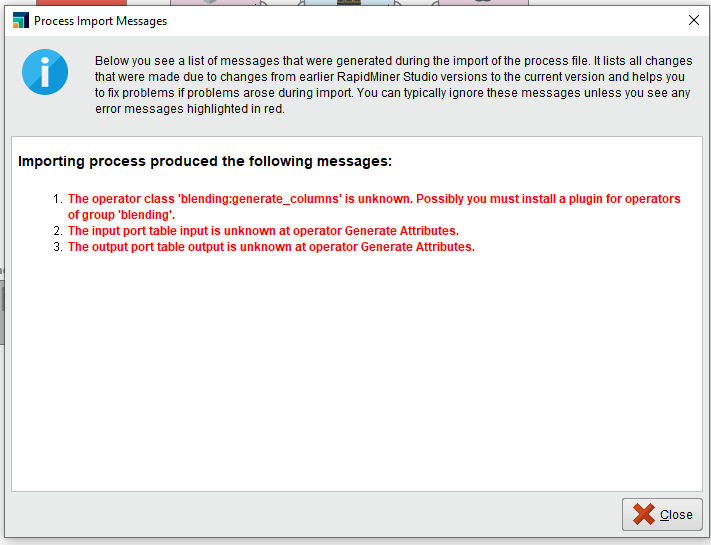• Member Posts: 59Guru
Hmm, that's a new one for me. What version of RapidMiner are you using? I built mine in v10.2. Just so you can keep working, here's a couple of screenshots of it: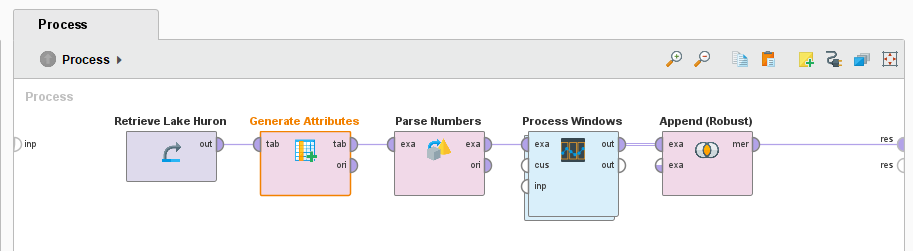Generate attributes has the following inside: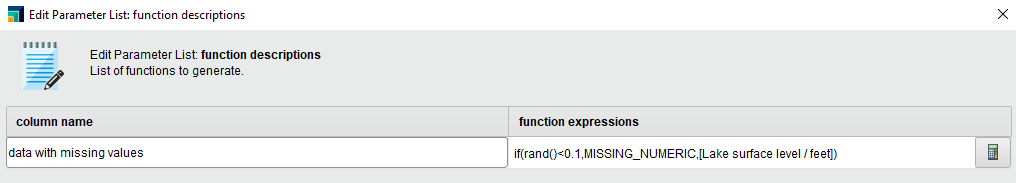These are the parameters of Process Windows: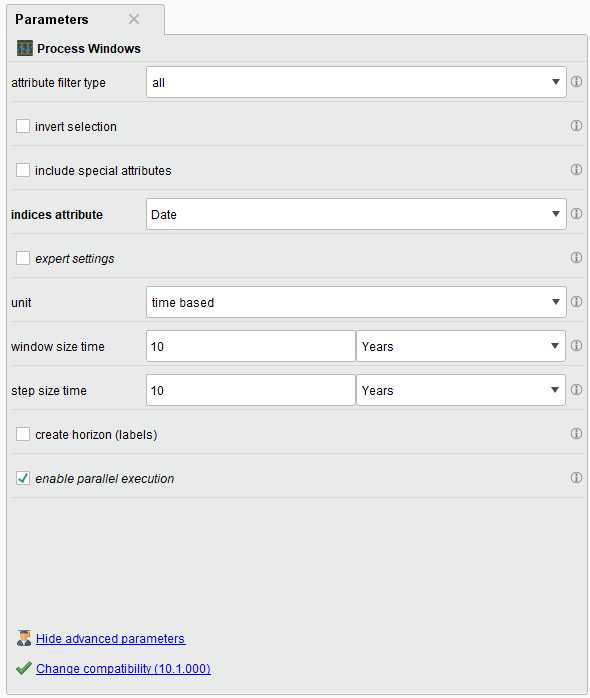And this is what you need inside Process Windows: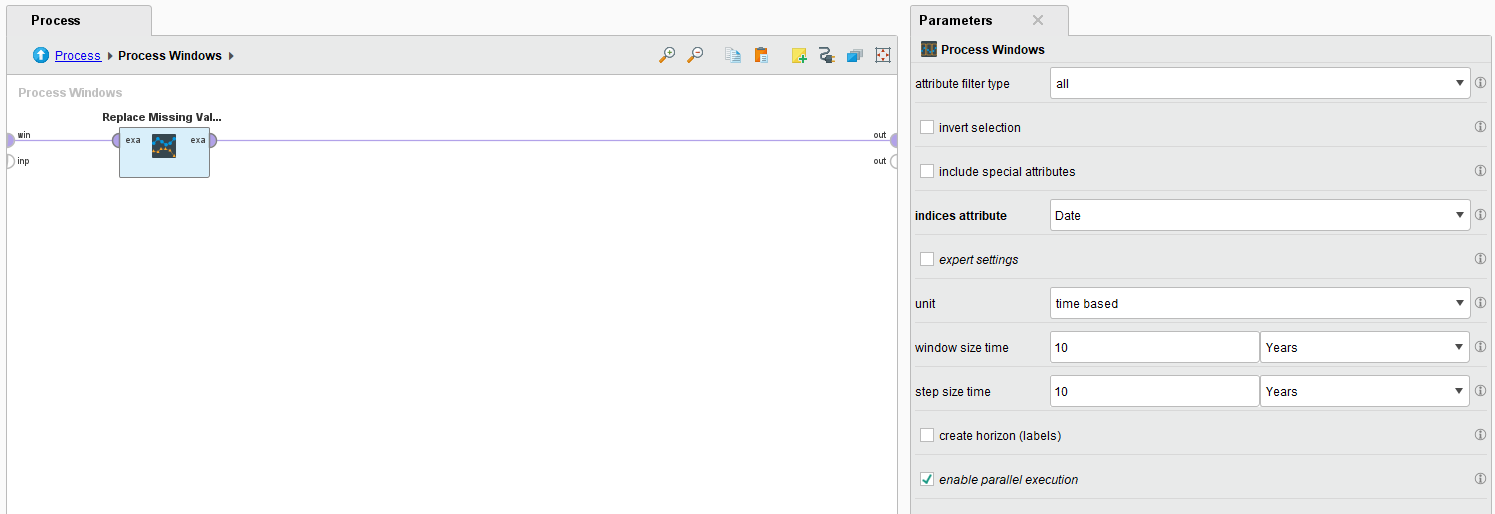You should find the Lake Heuron data in the training examples. Any problems, please do let me know. I'll report back if I figure out why it's not importing.
• Administrator, Moderator, Employee, Member Posts: 66RM Team Member
Hey, blending:generate_columns is the class associated with the core "Generate Attributes" operator. It should always be present in a regular installation. What version of RapidMiner Studio are you using?
• Member Posts: 5Newbie
edited September 26
I'm using version 9.10.
• Member Posts: 59Guru
If possible, and you don't have any dependent AI Hubs etc, I would try and update to the latest version. If not, I don't happen to have v9.10 on my machine but looking back the syntax is a little different. You could try replacing the relevant part of the xml process:
VERSION 10.2: <operator activated="true" class="blending:generate_columns" compatibility="10.2.000" expanded="true" height="82" name="Generate Attributes" origin="GENERATED_TUTORIAL" width="90" x="246" y="34">
VERSION 9.10: <operator activated="true" class="generate_attributes" compatibility="6.4.000" expanded="true" height="82" name="Generate Attributes" origin="GENERATED_SAMPLE" width="90" x="246" y="34">

From my side this sort of works, although needed to reconnect a couple of ports. Here's the whole thing again but which might now import successfully:

<?xml version="1.0" encoding="UTF-8"?><process version="10.2.000">
<context>
<input/>
<output/>
<macros/>
</context>
<operator activated="true" class="process" compatibility="10.2.000" expanded="true" name="Process" origin="GENERATED_TUTORIAL">
<parameter key="logverbosity" value="init"/>
<parameter key="random_seed" value="2001"/>
<parameter key="send_mail" value="never"/>
<parameter key="process_duration_for_mail" value="30"/>
<parameter key="encoding" value="SYSTEM"/>
<process expanded="true">
<operator activated="true" class="retrieve" compatibility="10.2.000" expanded="true" height="68" name="Retrieve Lake Huron" origin="GENERATED_TUTORIAL" width="90" x="112" y="34">
<parameter key="repository_entry" value="//Samples/Time Series/data sets/Lake Huron"/>
</operator>
<operator activated="true" class="generate_attributes" compatibility="6.4.000" expanded="true" height="82" name="Generate Attributes" origin="GENERATED_SAMPLE" width="90" x="246" y="34">
<list key="function_descriptions">
<parameter key="data with missing values" value="if(rand()&lt;0.1,MISSING_NUMERIC,[Lake surface level / feet])"/>
</list>
<parameter key="keep_all_columns" value="true"/>
</operator>
<operator activated="true" class="parse_numbers" compatibility="10.2.000" expanded="true" height="82" name="Parse Numbers" width="90" x="380" y="34">
<parameter key="attribute_filter_type" value="all"/>
<parameter key="attribute" value=""/>
<parameter key="attributes" value=""/>
<parameter key="use_except_expression" value="false"/>
<parameter key="value_type" value="nominal"/>
<parameter key="use_value_type_exception" value="false"/>
<parameter key="except_value_type" value="file_path"/>
<parameter key="block_type" value="single_value"/>
<parameter key="use_block_type_exception" value="false"/>
<parameter key="except_block_type" value="single_value"/>
<parameter key="invert_selection" value="false"/>
<parameter key="include_special_attributes" value="false"/>
<parameter key="decimal_character" value="."/>
<parameter key="grouped_digits" value="false"/>
<parameter key="grouping_character" value=","/>
<parameter key="infinity_representation" value=""/>
<parameter key="unparsable_value_handling" value="fail"/>
</operator>
<operator activated="false" class="time_series:windowing" compatibility="10.1.000" expanded="true" height="103" name="Windowing" width="90" x="179" y="340">
<parameter key="attribute_filter_type" value="all"/>
<parameter key="attribute" value="Date"/>
<parameter key="attributes" value=""/>
<parameter key="use_except_expression" value="false"/>
<parameter key="value_type" value="attribute_value"/>
<parameter key="use_value_type_exception" value="false"/>
<parameter key="except_value_type" value="time"/>
<parameter key="block_type" value="attribute_block"/>
<parameter key="use_block_type_exception" value="false"/>
<parameter key="except_block_type" value="value_matrix_row_start"/>
<parameter key="invert_selection" value="false"/>
<parameter key="include_special_attributes" value="true"/>
<parameter key="has_indices" value="false"/>
<parameter key="indices_attribute" value="Date"/>
<parameter key="sort_time_series" value="false"/>
<parameter key="expert_settings" value="false"/>
<parameter key="unit" value="time based"/>
<parameter key="windows_defined" value="from start"/>
<parameter key="custom_start_point" value="5"/>
<parameter key="custom_end_point" value="100"/>
<parameter key="window_size" value="20"/>
<parameter key="custom_start_time" value="2000-01-01 00:00:00"/>
<parameter key="custom_end_time" value="2030-01-01 00:00:00"/>
<parameter key="date_format" value="yyyy-MM-dd HH:mm:ss"/>
<parameter key="window_size_time" value="10.Years"/>
<parameter key="windows_stop_definition" value="from next window start"/>
<parameter key="window_start_attribute" value=""/>
<parameter key="window_stop_attribute" value=""/>
<parameter key="no_overlapping_windows" value="false"/>
<parameter key="step_size" value="1"/>
<parameter key="step_size_time" value="10.Years"/>
<parameter key="create_horizon_(labels)" value="false"/>
<parameter key="horizon_attribute" value=""/>
<parameter key="horizon_size" value="1"/>
<parameter key="horizon_offset" value="0"/>
<parameter key="horizon_size_time" value="1.Hours"/>
<parameter key="horizon_offset_time" value="0.Minutes"/>
<parameter key="horizon_start_attribute" value=""/>
<parameter key="horizon_stop_attribute" value=""/>
</operator>
<operator activated="true" class="time_series:process_windows" compatibility="10.1.000" expanded="true" height="103" name="Process Windows" width="90" x="514" y="34">
<parameter key="attribute_filter_type" value="all"/>
<parameter key="attribute" value=""/>
<parameter key="attributes" value=""/>
<parameter key="use_except_expression" value="false"/>
<parameter key="value_type" value="attribute_value"/>
<parameter key="use_value_type_exception" value="false"/>
<parameter key="except_value_type" value="time"/>
<parameter key="block_type" value="attribute_block"/>
<parameter key="use_block_type_exception" value="false"/>
<parameter key="except_block_type" value="value_matrix_row_start"/>
<parameter key="invert_selection" value="false"/>
<parameter key="include_special_attributes" value="false"/>
<parameter key="has_indices" value="false"/>
<parameter key="indices_attribute" value="Date"/>
<parameter key="sort_time_series" value="false"/>
<parameter key="expert_settings" value="false"/>
<parameter key="unit" value="time based"/>
<parameter key="windows_defined" value="from start"/>
<parameter key="custom_start_point" value="5"/>
<parameter key="custom_end_point" value="100"/>
<parameter key="window_size" value="20"/>
<parameter key="custom_start_time" value="2000-01-01 00:00:00"/>
<parameter key="custom_end_time" value="2030-01-01 00:00:00"/>
<parameter key="date_format" value="yyyy-MM-dd HH:mm:ss"/>
<parameter key="window_size_time" value="10.Years"/>
<parameter key="windows_stop_definition" value="from next window start"/>
<parameter key="window_start_attribute" value=""/>
<parameter key="window_stop_attribute" value=""/>
<parameter key="no_overlapping_windows" value="false"/>
<parameter key="step_size" value="1"/>
<parameter key="step_size_time" value="10.Years"/>
<parameter key="create_horizon_(labels)" value="false"/>
<parameter key="horizon_attribute" value=""/>
<parameter key="horizon_size" value="1"/>
<parameter key="horizon_offset" value="0"/>
<parameter key="horizon_size_time" value="1.Hours"/>
<parameter key="horizon_offset_time" value="0.Minutes"/>
<parameter key="horizon_start_attribute" value=""/>
<parameter key="horizon_stop_attribute" value=""/>
<parameter key="enable_parallel_execution" value="true"/>
<process expanded="true">
<operator activated="true" class="time_series:replace_missing_values" compatibility="10.1.000" expanded="true" height="68" name="Replace Missing Values (Series)" width="90" x="112" y="34">
<parameter key="attribute_filter_type" value="all"/>
<parameter key="attribute" value=""/>
<parameter key="attributes" value=""/>
<parameter key="use_except_expression" value="false"/>
<parameter key="value_type" value="attribute_value"/>
<parameter key="use_value_type_exception" value="false"/>
<parameter key="except_value_type" value="time"/>
<parameter key="block_type" value="attribute_block"/>
<parameter key="use_block_type_exception" value="false"/>
<parameter key="except_block_type" value="value_matrix_row_start"/>
<parameter key="invert_selection" value="false"/>
<parameter key="include_special_attributes" value="false"/>
<parameter key="has_indices" value="false"/>
<parameter key="indices_attribute" value=""/>
<parameter key="sort_time_series" value="false"/>
<parameter key="overwrite_attributes" value="true"/>
<parameter key="new_attributes_postfix" value="_cleaned"/>
<parameter key="replace_type_numerical" value="average"/>
<parameter key="replace_type_nominal" value="previous value"/>
<parameter key="replace_type_date_time" value="previous value"/>
<parameter key="replace_value_numerical" value="0.0"/>
<parameter key="replace_value_nominal" value="unknown"/>
<parameter key="skip_other_missings" value="true"/>
<parameter key="replace_infinity" value="true"/>
<parameter key="replace_empty_strings" value="true"/>
<parameter key="ensure_finite_values" value="true"/>
</operator>
<connect from_port="windowed example set" to_op="Replace Missing Values (Series)" to_port="example set"/>
<connect from_op="Replace Missing Values (Series)" from_port="example set" to_port="output 1"/>
<portSpacing port="source_windowed example set" spacing="0"/>
<portSpacing port="source_input 1" spacing="0"/>
<portSpacing port="sink_output 1" spacing="0"/>
<portSpacing port="sink_output 2" spacing="0"/>
</process>
</operator>
<operator activated="true" class="model_simulator:append_robust" compatibility="10.2.000" expanded="true" height="82" name="Append (Robust)" width="90" x="648" y="34"/>
<connect from_op="Retrieve Lake Huron" from_port="output" to_op="Generate Attributes" to_port="table input"/>
<connect from_op="Generate Attributes" from_port="table output" to_op="Parse Numbers" to_port="example set input"/>
<connect from_op="Parse Numbers" from_port="example set output" to_op="Process Windows" to_port="example set"/>
<connect from_op="Process Windows" from_port="output 1" to_op="Append (Robust)" to_port="example set 1"/>
<connect from_op="Append (Robust)" from_port="merged set" to_port="result 1"/>
<portSpacing port="source_input 1" spacing="0"/>
<portSpacing port="sink_result 1" spacing="0"/>
<portSpacing port="sink_result 2" spacing="0"/>
</process>
</operator>
</process>